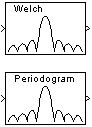# Periodogram

Power spectral density or mean-square spectrum estimate using periodogram method

• Library:
• DSP System Toolbox / Estimation / Power Spectrum Estimation

•## Description

The Periodogram block estimates the power spectral density (PSD) or mean-square spectrum (MSS) of the input. The block uses the periodogram method and Welch's averaged, modified periodogram method. The block averages the squared magnitude of the FFT function computed over windowed sections of the input. It then normalizes the spectral average by the square of the sum of the window samples. For more information, see Periodogram and Welch's Method.

## Ports

### Input

expand all

Input signal, specified as a vector or a matrix.

The block treats M-by-N matrix input as M sequential time samples from N independent channels.

Data Types: `single` | `double`
Complex Number Support: Yes

### Output

expand all

Output signal, returned as a vector or a matrix.

Each column of the output matrix contains the estimate of the power spectral density of the corresponding input column at Nfft equally spaced frequency points. The frequency points are in the range [0,Fs), where Fs is the sampling frequency of the signal.

The block computes a separate estimate for each of the N independent channels and generates an Nfft-by-N matrix output.

Data Types: `single` | `double`

## Parameters

expand all

Specify the type of measurement for the block to perform: ```Power spectral density``` or ```Mean-square spectrum```.

Tunable: No

Select the type of window to apply.

For details, see the Window Function block reference page.

Tunable: Yes

Enter the β parameter for the Kaiser window. Increasing Beta widens the mainlobe and decreases the amplitude of the sidelobes in the displayed frequency magnitude response.

For more details, see the Window Function block reference page.

Tunable: Yes

#### Dependencies

This parameter becomes visible if, for the `Window` parameter, you chose `Kaiser`.

From the list, choose `Symmetric` or `Periodic`.

See the Window Function block reference page for more details.

Tunable: Yes

#### Dependencies

This parameter becomes visible only if you set the `Window` parameter to one of `Blackman`, `Hamming`, `Hann`, or `Hanning`.

Set this parameter to `FFTW` to support an arbitrary length input signal. The block restricts generated code with an FFTW implementation to MATLAB® host computers.

Set this parameter to `Radix-2` for bit-reversed processing, fixed or floating-point data, or for portable C-code generation by using Simulink® Coder™. The first dimension M of the input matrix must be a power of two. To work with other input sizes, use the Pad block to pad or truncate these dimensions to powers of two, or if possible, choose the FFTW implementation.

Set this parameter to `Auto` to enable the block choose the FFT implementation. For non-power-of-two transform lengths, the block restricts generated code to MATLAB host computers.

Tunable: No

Enter the level, in decibels (dB), of stopband attenuation, Rs, for the Chebyshev window.

Tunable: Yes

#### Dependencies

This parameter becomes visible if, for the `Window` parameter, you choose `Chebyshev`.

When you select this check box, the block uses the input frame size as the number of data points, Nfft, on which to perform the FFT. To specify the number of points on which to perform the FFT, clear the Inherit FFT length from input dimensions check box. You can then specify a power of two FFT length by using the FFT length parameter.

Tunable: No

Enter the number of data points on which to perform the FFT, Nfft. When Nfft is larger than the input frame size, the block zero-pads each frame as needed. When Nfft is smaller than the input frame size, the block wraps each frame as needed.

When you set the `FFT implementation` parameter to `Radix-2`, this value must be a power of two.

Tunable: No

#### Dependencies

This parameter becomes visible only when you clear the Inherit FFT length from input dimensions check box.

Specify the number of spectral to average. When you set this value to `1`, the block computes the periodogram of the input. When you set this value greater `1`, the block implements Welch's Method to compute a modified periodogram of the input.

Tunable: No

If you select the Inherit sample time from input check box, the block computes the frequency data from the sample period of the input signal. For the block to produce valid output, the following conditions must hold:

• The input to the block is the original signal, with no samples added or deleted (by insertion of zeros, for example).

• The sample period of the time-domain signal in the simulation equals the sample period of the original time series.

If these conditions do not hold, clear the Inherit sample time from input check box. You can then specify a sample time by using the `Sample time of original time series` parameter.

#### Dependencies

This parameter becomes visible only if you set the `Measurement` parameter to ```Power spectral density```.

Specify the sample time of the original time-domain signal.

Tunable: No

#### Dependencies

This parameter becomes visible only when you clear the `Inherit sample time from input` check box.

## Block Characteristics

 Data Types `double` | `single` Multidimensional Signals `No` Variable-Size Signals `No`

 Frigo, M. and S. G. Johnson, “FFTW: An Adaptive Software Architecture for the FFT,” Proceedings of the International Conference on Acoustics, Speech, and Signal Processing , Vol. 3, 1998, pp. 1381-1384.

 Oppenheim, A. V. and R. W. Schafer. Discrete-Time Signal Processing . Englewood Cliffs, NJ: Prentice Hall, 1989.

 Orfanidis, S. J. Introduction to Signal Processing . Englewood Cliffs, NJ: Prentice-Hall, 1995.

 Proakis, J. and D. Manolakis. Digital Signal Processing. 3rd ed. Englewood Cliffs, NJ: Prentice-Hall, 1996.

Watch now